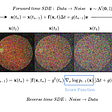# Variational Autoencoders with Tensorflow Probability Layers

Posted by Ian Fischer, Alex Alemi, Joshua V. Dillon, and the TFP Team

At the 2019 TensorFlow Developer Summit, we announced TensorFlow Probability (TFP) Layers. In that presentation, we showed how to build a powerful regression model in very few lines of code. Here, we will show how easy it is to make a Variational Autoencoder (VAE) using TFP Layers.

# TensorFlow Probability Layers

TFP Layers provides a high-level API for composing distributions with deep networks using Keras. This API makes it easy to build models that combine deep learning and probabilistic programming. For example, we can parameterize a probability distribution with the output of a deep network. We will use this approach here.

# Variational Autoencoders and the ELBO

Variational Autoencoders (VAEs) are popular generative models being used in many different domains, including collaborative filtering, image compression, reinforcement learning, and generation of music and sketches.

In the traditional derivation of a VAE, we imagine some process that generates the data, such as a latent variable generative model. Consider the process of drawing digits, as in MNIST. Suppose that before you draw the digit, you first decide which digit you will draw, imagining some fuzzy picture in your head. Then, you put pen to paper and try to create the picture in the real world. We can formalize this two step process:

1. You sample some latent representation z from some prior distribution z ~ p(z). This is the fuzzy picture in your head — let’s say of a “3”.
2. Based on your sample, you draw the actual picture representation x, modeled itself as a stochastic process x ~ p(x|z). This captures the idea that each time you write a “3”, it looks at least a little different.

Thus, when a handwritten digit is created, we imagine some of the variation is due to some kind of signal inherent to the process, such as the class identity of the MNIST digit, and some of that variation is due to noise, such as differences in the precise angles of the lines among different samples of the same digit drawn by the same person. Broadly, a VAE is an attempt to try to separate the signal from the noise with an explicit model of both processes.

To train this objective, we maximize the ELBO (Evidence Lower BOund) objective:

Where the three probability density functions are:

• p(z), the prior on the latent representation z,
• q(z|x), the variational encoder, and
• p(x|z), the decoder — how likely is the image x given the latent representation z.

The ELBO is a lower bound on log p(x), the log probability of an observed data point. The first integral in the ELBO equation is the reconstruction term. It asks how likely we are to start at an image x, encode it to z, decode it, and get back the original x. The second term is the KL divergence term. It measures how close together our encoder and prior are; you can think of this term as just trying to keep our encoder honest. If our encoder generates z samples that are too unlikely given our prior, the objective is worse than if it generates z samples more typical of the prior. Thus, the encoder should differ from the prior only if the cost of doing so is outweighed by the benefit in the reconstruction term.

# Code

From the description above, we can see that it is natural to model three different components individually: the prior p(z), the variational encoder q(z|x), and the decoder p(x|z). You can follow along by running this colab, which trains a VAE on MNIST in a few minutes on a cloud GPU.

# Prior

The simplest prior typically used in a VAE is an isotropic Gaussian:

Here, we have just created a TFP independent Gaussian distribution with no learned parameters, and we have specified that our latent variable, z, will have 16 dimensions.

# Encoder

For our encoder distribution, we will use a full-covariance Gaussian distribution, with its mean and covariance matrices parameterized by the output of a neural network. This may sound complicated, but it is very easy to express with TFP Layers:

The encoder is just a normal Keras Sequential model, consisting of convolutions and dense layers, but the output is passed to a TFP Layer, `MultivariateNormalTril()`, which transparently splits the activations from the final `Dense()` layer into the parts needed to specify both the mean and the (lower triangular) covariance matrix, the parameters of a Multivariate Normal. We used a helper, `tfpl.MultivariateNormalTriL.params_size(encoded_size)`, to make the `Dense()` layer output the correct number of activations (i.e., the distribution’s parameters). Finally, we said that the distribution should contribute a “regularization” term to the final loss. Specifically, we are adding the KL divergence between the encoder and the prior to the loss, which is the KL term in the ELBO that we described above. (Fun fact: we can turn this VAE into a β-VAE simply by changing the `weight` argument to something other than 1!)

# Decoder

For our decoder, we will use a simple “mean-field decoder”, which in this case will be a pixel-independent Bernoulli distribution:

The form here is essentially the same as the encoder, but now we are using transposed convolutions to take our latent representation, which is a 16 dimensional vector, and turn it into a 28 x 28 x 1 tensor. That final tensor parameterizes the pixel-independent Bernoulli distribution.

# Loss

We are now ready to build the full model and specify the rest of the loss function.

Our model is just a Keras Model where the outputs are defined as the composition of the encoder and the decoder. Since the encoder already added the KL term to the loss, we need to specify only the reconstruction loss (the first term of the ELBO above).

The loss function takes two arguments — the original input, x, and the output of the model. We call that `rv_x` because it is a random variable. This example demonstrates some of the core magic of TFP Layers — even though Keras and Tensorflow view the TFP Layers as outputting tensors, TFP Layers are actually Distribution objects. Thus, we can make our loss function be the negative log likelihood of the data given the model: `-rv_x.log_prob(x)`.

# Wait, what?

It is worth taking a moment to understand what TFP Layers is actually doing to integrate transparently with Keras. As we said, the output of a TFP Layer is actually a Distribution object. We can check that with the following code:

But if a TFP Layer returns a Distribution, what happens when we compose the decoder with the output of the encoder: `decoder_model(encoder_model.outputs))`? Well, in order for Keras to view the encoder distribution as a Tensor, TFP Layers actually “reifies” the distribution as a sample from that distribution, which is just a fancy way of saying that Keras sees the Distribution object as the Tensor we would have gotten, had we called `encoder_model.sample()`. But, when we need to access the Distribution object directly, we can — just like we do in the loss function when we call `rv_x.log_prob(x)`. TFP Layers provides the distribution-like and Tensor-like behaviors automatically, so you don’t need to worry about Keras getting confused.

# Training

Training the model is as easy as training any Keras model: we just call `vae_model.fit()`:

With this model, we are able to get an ELBO of around 115 nats (the nat is the natural logarithm equivalent of the bit — 115 nats is around 165 bits). Of course, this performance isn’t state-of-the-art, but it is easy to make any of the three components more powerful starting from this basic setup. Also, it already generates nice looking digits!

# Summary

In this blog post, we demonstrated how to combine deep learning with probabilistic programming: we built a variational autoencoder that used TFP Layers to pass the output of a Keras Sequential model to a probability distribution in TFP.

We utilized the tensor-like and distribution-like semantics of TFP layers to make our code relatively straightforward.

--

--

--

## More from TensorFlow

TensorFlow is an end-to-end open source platform for machine learning.

## Machine Learing : K-Nearest Neighbors (Theory Explained)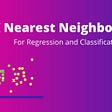## Paper Review on Single Shot Detector (SSD)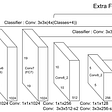## Deep Learning for Air Quality Prediction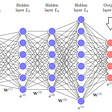## Human-Centric & Interpretable Machine Learning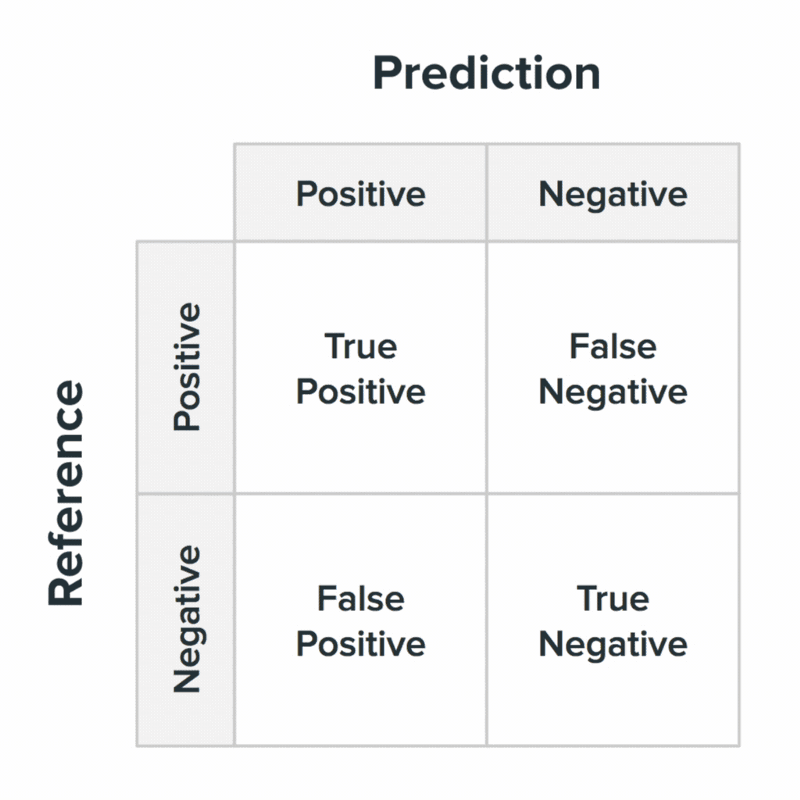## Simplified Quantum Machine Learning (QML) Classification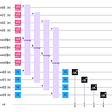## Practical Tips for Training a Music Model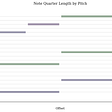## TFDevSummit2019: Top 5 TensorFlow Announcements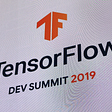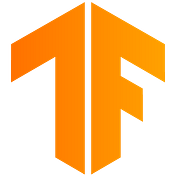## TensorFlow

TensorFlow is a fast, flexible, and scalable open-source machine learning library for research and production.

## Variational Autoencoders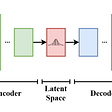## TPU is not always faster than GPU #short## Why over-parameterised deep nets don’t overfit?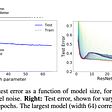## A New Class of Generative Models